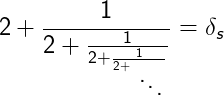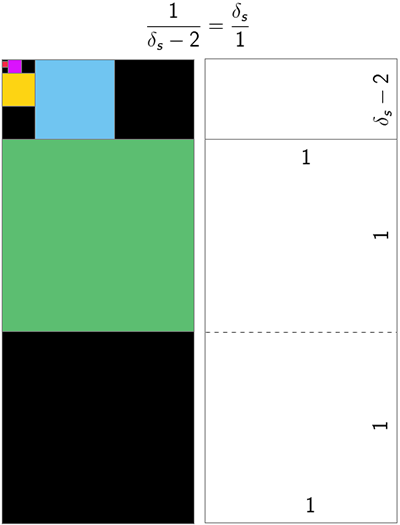# Silver Ratio Calculator

You can use this Silver Ratio Calculator to quickly and easily use the Silver Ratio to identify a missing value.

How to use the calculator:

1. Input either the width (A) or the length (B).
2. Click on the "Calculate" button to compute the missing value.
Silver Ratio Calculator

Enter only one value in any of the fields below:

You may set the number of decimal places in the online calculator. By default there are only two decimal places.

Results
• A =
• B =

## Reference

The silver ratio, which is typically represented by δs, operates in a similar manner to the renowned golden ratio, and it is used in a variety of geometric and mathematical expressions.

The aspect ratio of a silver rectangle is 1:1+√2, or roughly 1:2.41421356.

The following continued fraction converges to δs:It is possible to resolve this arithmetic expression by solving the following equation: x = 2 + 1/x

Furthermore, the silver ratio appears as the limiting ratio of the solutions to the Pell equation: 2x2 ± 1 = y2

For example, the first few solution pairs are as follows:

(1, 1)   2x2 − 1 = y2

(2, 3)   2x2 + 1 = y2

(5, 7)   2x2 − 1 = y2

(12, 17)   2x2 + 1 = y2

(29, 41)   2x2 − 1 = y2

(70, 99)   2x2 + 1 = y2

(169, 239)   2x2 − 1 = y2

(408, 577)   2x2 + 1 = y2

(985, 1393)   2x2 − 1 = y2

(2378, 3363)   2x2 + 1 = y2

...

If you apply the ratio of the consecutive y terms, you have the following:

3/1 = 3

7/3 = 2.333333

17/7 = 2.428571

41/17 = 2.411765

99/41 = 2.414634

577/239 = 2.414225

3363/2378 = 2.414213

As the above reveals, the ratio converges to 1+√2. A similar pattern will emerge if you apply the ratio of the sequential x terms.

To comprehend δs from a geometric perspective, let's say you have a rectangle of which the sides are in a ratio of 1:δs. When you remove two perfect squares from the rectangle, the remaining rectangle is referred to as a silver rectangle. Repeating the process will result in the following pattern:You may also be interested in our Ratio Calculator or Aspect Ratio Calculator